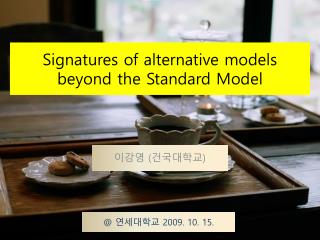DownloadDownload PresentationSignatures of alternative models beyond the Standard Model

# Signatures of alternative models beyond the Standard Model

Télécharger la présentation## Signatures of alternative models beyond the Standard Model

- - - - - - - - - - - - - - - - - - - - - - - - - - - E N D - - - - - - - - - - - - - - - - - - - - - - - - - - -
##### Presentation Transcript

1. Signatures of alternative models beyond the Standard Model 이강영 (건국대학교) @ 연세대학교 2009. 10. 15.

2. Contents • Introduction • Z’ • W’ • H+ • H++ • t’, b’ • Q, L • Dark matter • Summary

3. Introduction • LHC will explore for the first time a relevant energy range, well above the Fermi scale. • LHC is the Energy frontier machine best to search for the new (heavy) particles. • We concentrate on the new particles discovery. • The detailed phenomenology depends upon the model.

4. LHC Thevoyage to the ultimate world • 2008년 9월 10일 공식적으로 가동. • 올 11월부터 정식가동. • 둘레 27km에 이르는 • 지구상 최대의 실험장치. 다음과 같은 세계를 탐구하는 양성자-양성자 충돌장치. ECM = 14 TeV (max) ~ v = 0.999999991c (c=빛의속도) ~ d = 0.000000000000000000014 m

5. Z’

6. Underlying Physics • Z’ : Extra neutral gauge boson • Exists when there is extra gauge symmetries. • U(1) extensions of the SM • LR model • Other gauge extended models • Other species : excited states of Z • Little Higgs model • Extra dimensional models

7. Examples of U(1) extensions E6 models breaking chain χ model : β=0 ψ model : β=π/2 η model : β=arctan(-√5/3)

8. LR model (WL3, WR3, B) basis Diagonalized to give eigenvalues

9. and eigenstates where Note that Unknown model parameters are MZ’ Z-Z’ mixing angle

10. Z’ couplings to quarks and leptons Lagrangian for a Z’ inE6 model The Z-Z’ mixing angle is given by

11. Phenomenology Z’ Drell-Yan process

12. Experimental limits PDG 2008

13. CDF collab., Phys. Rev. Lett., 95, 252001 (2005)

14. Identification of Z’ usingt and b e.g. S. Godfrey and T. A. W. Martin, Phys. Rev. Lett., 101, 151803 (2008) Kq depends on QCD and EW corrections.

15. S. Godfrey and T. A. W. Martin, PRL 101, 151803 (2008)

16. Measuring Z’ couplings at the LHC e.g. F. Petriello and S. Quackenbush, Phys. Rev. D 77, 115004 (2008). • Z’ mass and totalwidth • Cross section to • Forward-backward asymmetry • Rapidity ratio • Off-peak asymmetry Basic Observables

17. Forward-backward asymmetry where y1 is introduced to exclude low Z’ rapidity events. • Rapidity ratio

18. M. Dittmar, Phys. Rev. D 55, 161 (1997)

19. Acceptance Cuts • Detector resolution effects are ignored. • Reconstruction efficiency of Z’ production is near 90% from CMS simulation. • CTEQ 6.5 NLO PDF used. • Integrated luminosity 100fb-1 unless stated otherwise. • Factorization and renormalization scale : MZ’

20. Calculation Differential cross section Parity symmetric couplings Parity violating couplings

21. Rewrite the differential cross section Absorbing We have

22. Define four observables

23. which are expressed in terms of observables

24. e.g. If we let Solving the Master equation to have

25. Results

26. If MZ’ =1.5 TeV, 100 fb-1 luminosity and y1=0.8 can discriminate the example models with 90% C.L. and 1 ab-1 luminosity (SLHC) will provide precise determination. • If MZ’ =3 TeV, 100 fb-1 luminosity and y1=0.4 can discriminate some models. • For MZ’ =3 TeV, 1 ab-1 luminosity (SLHC) will provide reasonable determination.

27. Exotic Z’ • Generation-dependent couplings • Leptophobic • Hadrophobic • Flavour-violating • And more…

28. W’

29. Underlying Physics • W’ : Extra charged gauge boson • Exists when there are extra gauge symmetries more than U(1). • LR model • Other gauge extended models • Other species : excited states of W • Little Higgs model • Extra dimensional models

30. LR model (e.g.) (WL+, WR+) basis Diagonalized to give where

31. Search for W’ W’ → l-ν • High energy single lepton final states • Single top production W’ → t b

32. Transverse mass • Edges of transverse mass distribution are crucially related to the mass of W’. D0 collaboration, PRL 100, 031804 (2008)

33. Experimental limits PDG 2008

34. CDF constraints

35. D0 observations D0 collaboration, PRL 100, 031804 (2008)

36. Feasibility of W’ at the CMS e.g. C. Hof, Acta. Phys. Pol. B 38, 443 (2007) Reference models : same couplings as the SM

37. Exotic W’ • Left-right asymmetric : coupling constants and CKM • Leptophobic • Hadrophobic • Flavour-dependent SU(2) • Exotic gauge self-couplings : W’-W-Z, W’-W’-Z … • And more…

38. H+

39. Underlying Physics • H+: Charged scalar • Exists when there is extra Higgs sector more than SM singlet. • 2HD model • MSSM and more extensions (NMSSM etc.) • LR model • Other GUT-based model